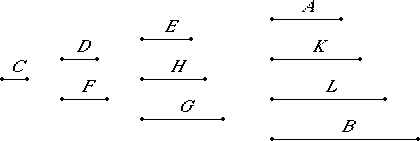# Proposition 10

If numbers fall between two numbers and a unit in continued proportion, then however many numbers fall between each of them and a unit in continued proportion, so many also fall between the numbers themselves in continued proportion.

Let the numbers D and E and the numbers F and G respectively fall between the two numbers A and B and the unit C in continued proportion.

I say that, as many numbers have fallen between each of the numbers A and B and the unit C in continued proportion as fall between A and B in continued proportion.

Multiply D by F to make H, and multiply the numbers D and F by H to make K and L respectively.VII.Def.20

Now, since the unit C is to the number D as D is to E, therefore the unit C measures the number D the same number of times as D measures E. But the unit C measures the number D according to the units in D, therefore the number D also measures E according to the units in D. Therefore D multiplied by itself makes E.

Again, since C is to the number D as E is to A, therefore the unit C measures the number D the same number of times as E measures A. But the unit C measures the number D according to the units in D, therefore E also measures A according to the units in D. Therefore D multiplied by E makes A.

For the same reason also F multiplied by itself makes G, and multiplied by G makes B.

VII.17

And, since D multiplied by itself makes E and multiplied by F makes H, therefore D is to F as E is to H.

VII.18

For the same reason also D is to F as H is to G. Therefore E is to H as H is to G.

VII.17

Again, since D multiplied by the numbers E and H makes A and K respectively, therefore E is to H as A is to K. But E is to H as D is to F, therefore D is to F as A is to K.

Again, since the numbers D and F multiplied by H make K and L respectively, therefore D is to F as K is to L. But D is to F as A is to K, therefore A is to K as K is to L. Further, since F multiplied by the numbers H and G makes L and B respectively, therefore H is to G as L is to B.

But H is to G as D is to F, therefore D is to F as L is to B. But it was also proved that D is to F as A is to K and as K is to L, therefore A is to K as K is to L and as L is to B. Therefore A, K, L, and B are in continued proportion.

Therefore, as many numbers as fall between each of the numbers A and B and the unit C in continued proportion, so many also fall between A and B in continued proportion.

Therefore, if numbers fall between two numbers and a unit in continued proportion, then however many numbers fall between each of them and a unit in continued proportion, so many also fall between the numbers themselves in continued proportion.

Q.E.D.

## Guide

Essentially, the proposition says that if a and b are (n–1)st powers of d and f, respectively, then the sequence

dn-1, dn-2f, dn-3f 2, ... , df n-2, f n-1

is in continued proportion.

This is a partial converse to the previous proposition; it doesn’t require that the generating numbers d and f be relatively prime.

The next two propositions are special cases of this proposition when the powers are squares and cubes.